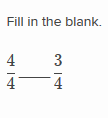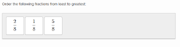FANDOM

1,895 Pages

The Comparing fractions with the same denominator exercise appears under the 3rd grade (U.S.) Math Mission. This exercise has users compare fractions in which the denominators are the same.

Types of Problems

There are three types of problems in this exercise:

1. Select the correct inequality symbol: This problem has two fractions and asks the user to select which inequality symbol, either < or >, can be put between them to make a true inequality.Select the correct inequality symbol
2. Put the fractions in increasing order: The dragging problem has three fractions that are to be placed in increasing numerical order.Put the fractions in increasing order

Strategies

This exercise is easy to get accuracy badges and speed badges because all three problem types can be done quickly and it is easy to maneuver in the interface.

1. In all cases since the denominator is the same users can answer the problem by concentrating on the numerators.
2. With common denominators, the larger numerator implies a larger fraction.
3. In some cases it will take a while for the fraction tiles to load and may cause users to lose their speed streak.

Real-life Applications

1. Fractions appear in every-day media to display information to consumers.
2. Fractions are used in real life in lots of different ways, but are commonly used in cooking, construction and science.
Community content is available under CC-BY-SA unless otherwise noted.